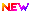Latest Applications Open 2023:JEE Advanced 2023 Mathematics Syllabus has been Released. The Authority has released JEE Advanced Mathematics Syllabus 2023 for the current academic year. Those candidates who only want to check the Mathematics syllabus are requested to check the complete Syllabus here, and also PDF is available for free Download.

JEE Advanced syllabus which contains the Subjects like Physics, Chemistry, and Mathematics which is given here Unit and section-wise in a distributed manner. JEE Main & JEE Advanced syllabus is a bit different from JEE Main 2023.

The contains are the same, but the difficulty level is high. Hence Candidates are requested to first check the JEE Advanced Eligibility and JEE Advance Exam Pattern.

## JEE Advanced 2023 Syllabus- PDF AvailableJEE Advanced 2023 Mathematics Syllabus has been released. Click Here to Download the PDF.

## JEE Advanced Section Wise Syllabus

Latest Applications For Various UG & PG Courses Open 2023

1. Parul University, Gujarat | Admissions Open for All Courses 2023. Apply Now
2. Manipal University, Karnataka | Admissions Open for All Courses 2023. Apply Now
3. Lovely Professional University, Punjab | Management Admissions Closing Soon! Apply Now
4. Alliance University, Bangalore | Admissions Open for All Courses 2023. Apply Now
5. NIMS University, Jaipur | Admissions Open for All Courses 2023. Apply Now
6. MIT WPU, Pune | Admissions Open for All Courses 2023. Apply Now
7. Chandigarh University, Punjab | Admissions Open for All Courses 2023. Apply Now
8. UPES, Dehradun | Admissions Open for All Courses 2023. Apply Now
9. Bennett University, Noida| Admissions Open for All Courses 2023. Apply Now

Latest Applications For Various UG & PG Courses Open 2023

1. Parul University, Gujarat | Admissions Open for All Courses 2023. Apply Now
2. Manipal University, Karnataka | Admissions Open for All Courses 2023. Apply Now
3. Lovely Professional University, Punjab | Management Admissions Closing Soon! Apply Now
4. Alliance University, Bangalore | Admissions Open for All Courses 2023. Apply Now
5. NIMS University, Jaipur | Admissions Open for All Courses 2023. Apply Now
6. MIT WPU, Pune | Admissions Open for All Courses 2023. Apply Now
7. Chandigarh University, Punjab | Admissions Open for All Courses 2023. Apply Now
8. UPES, Dehradun | Admissions Open for All Courses 2023. Apply Now
9. Bennett University, Noida| Admissions Open for All Courses 2023. Apply Now
 Algebra Algebra of complex numbers, addition, multiplication, conjugation, polar representation, properties of modulus and principal argument, triangle inequality, cube roots of unity, geometric interpretations. Quadratic equations with real coefficients, relations between roots and coefficients, the formation of quadratic equations with given roots, symmetric functions of roots. Arithmetic, geometric and harmonic progressions, arithmetic, geometric and harmonic means, sums of finite arithmetic and geometric progressions, infinite geometric series, sums of squares and cubes of the first n natural numbers. Logarithms and their properties. Permutations and combinations, binomial theorem for a positive integral index, properties of binomial coefficients. Matrices Matrices as a rectangular array of real numbers, equality of matrices, addition, multiplication by a scalar and product of matrices, transpose of a matrix, Determinant of a square matrix of order up to three, inverse of a square matrix of order up to three, properties of these matrix operations, diagonal, symmetric and ask Probability Addition and multiplication rules of probability, conditional probability, Bayes Theorem, independence of events, computation of the probability of events using permutations and combinations. Trigonometry Addition and multiplication rules of probability, conditional probability, Bayes Theorem, independence of events, computation of the likelihood of events using permutations and combinations. Analytical Geometry Two dimensions: Cartesian coordinates, the distance between two points, section formulae, the shift of origin. The equation of a straight line in various forms, angle between two lines, the distance of a point from a line; Lines through the point of intersection of two given lines, the equation of the bisector of the angle between two lines, concurrency of lines; Centroid, orthocentre, incentre, and circumcentre of a triangle. The equation of a circle in various forms, equations of tangent, normal and chord. Parametric equations of a circle, the intersection of a circle with a straight line or a circle, the comparison of a circle through the points of intersection of two circles and those of a circle and a straight line. Equations of a parabola, ellipse, and hyperbola in standard form, their foci, directrices and eccentricity, parametric equations, equations of tangent and normal. Locus problems. Three dimensions: Direction cosines and direction ratios, the equation of a straight line in space, the equation of a plane, a distance of a point from a plane. Differential Calculus Real-valued functions of a real variable, into, onto and one-to-one functions, sum, difference, product and quotient of two functions, composite functions, absolute value, polynomial, rational, trigonometric, exponential and logarithmic functions. Limit and continuity of a function, limit, and continuity of the sum, difference, product and quotient of two functions, L’Hospital rule of evaluation of limits of functions. Even and odd functions, the inverse of a function, continuity of composite functions, the intermediate value property of continuous functions. A derivative of a function, a derivative of the sum, difference, product and quotient of two functions, chain rule, derivatives of polynomial, rational, trigonometric, inverse trigonometric, exponential and logarithmic functions. Derivatives of implicit functions, derivatives up to order two, geometrical interpretation of the derivative, tangents, and normals, increasing and decreasing functions, maximum and minimum values of a function, Rolle’s theorem and Lagrange’s mean value theorem. Integral calculus Integration as the inverse process of differentiation, indefinite integrals of standard functions, definite integrals and their properties, fundamental theorem of integral calculus. Integration by parts, integration by the methods of substitution and partial fractions, application of definite integrals to the determination of areas involving simple curves. Formation of ordinary differential equations, a solution of homogeneous differential equations, separation of variables method, linear first-order differential equations. Vectors Addition of vectors, scalar multiplication, dot and cross products, scalar triple products and their geometrical interpretations.

For further queries regarding JEE Advanced Mathematics Syllabus 2023, you may leave your queries below.

1.Natvarparmar says:

Your 2020 board exam paper solutionManipal UniversityLPUAlliance UniversityNIMS UniversityMIT WPUChandigarh UniversityUPESBennett University